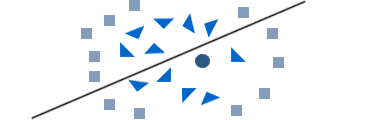# Support Vector Machine (Statistics)

The master bioinformatics offers the chance to get acquainted to several machine learning techniques. Machine learning algorithms are used in various courses, the theoretical ground work is taught in statistics. There we repeat how linear regression and hidden markov machines work, learning more about the mathematical concept of markov chains, and we get introduced to support vector machines (SVMs) and how to make them more efficient using the kernel trick.

SVMs are supervised learning models usually used on very large data sets which you want to divide into two classes. The division is performed by laying a plane or hyperplane between the two classes and maximizing the distance to each of them. If the classes are not linearly separable you have to transform the input space with a transformation function, called phi here, which introduces more dimensions. This function phi has to be cleverly chosen such that the two classes get cleanly separated without introducing too many new dimensions. Of course if you add enough dimensions everything can become separable, even if in reality there are no features distinguishing them. Another problem with adding too many dimensions is overfitting (learning properties of single data points instead of general class properties). Even when it does make sense to introduce this many new dimensions you will end up running into the curse of dimensionality. For with every new dimension the input points end up further apart from each other until the data is so sparse that the positioning of the dividing hyper plane becomes in a way arbitrary, which of course compromises your results when trying to classify new points.

##### Match the functions used for transforming the input space to the resulting decision borders. x=(x1,x2)

Φ(x): ℝ2 → ℝ2

We didn't change the number of dimensions, the space is still two-dimensional and thus the decision boundary linear.

Φ(x): ℝ2 → ℝ5

Enough dimensions were added to correctly divide the two classes, but not overfit.

Φ(x): ℝ2 → ℝ102

So many dimensions were added that the decision boundary was fitted to single points of one class.

1.2.3.You get feedback for each answer by clicking on the button.

Imagine that the points represent patients with a certain disease which we want to classify by measuring certain biomarkers. Squares indicate healthy patients, triangles diseased patiens. Now we want to predict if the patient marked with the circle is sick.

##### Mark if the following statements are true or not.
richtig
falsch

The new patient can be classified correctly with a model like model 1.

It can happen but it would be due to chance, not due to a good model.

Model 1 shouldn't be trusted because of the curse of dimensionality.

We didn't change the number of dimensions yet.

We should use the most simple model which still explains the data correctly.

That is generally what we do in science (see Ockham's razor).

Raising the number of dimension above 50 will never give good results.

In most problems this would be overkill, but if you have a lot of explaining features and a huge amount of data available it might be necessary and doable.

The decision border can take any form in the transformed higher-dimensional space.

In the dimension we introduce the decision border in it is always a hyperplane.

The decision border can take any form in the original space.

If you introduce an unimaginably big amount of new dimensions and thereby transformations you can achieve any form you would like.

You get feedback for each answer by clicking on the button.